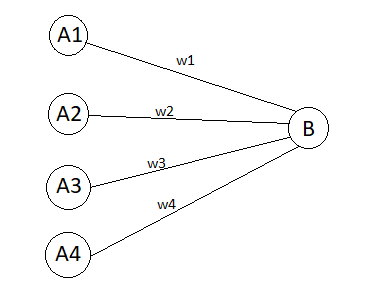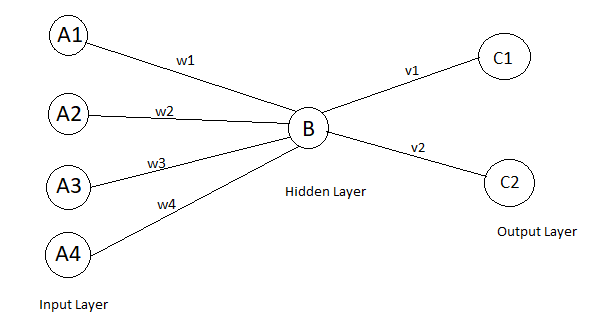## Introduction to Neural Networks

### What are Neural Networks?

Artificial neural network is an information processing system which is inspired by biological neural network or neural biology.Neural nets are used to solve various problems.It can be applied to storing and recalling data or patterns, classifying patterns, performing the mapping from input patterns to output patterns, identifying and grouping similar patterns.
A neural net has a large number of simple processing units called neurons, cells or nodes.Each neuron is connected to another neuron by directed links which have an associated weight to it. When a signal passes through links, it gets multiplied by their respective weights.A neuron is connected with multiple neurons, all the signals are added up and then there is an activation function which checks if the added signal is greater than some threshold value, if it is greater then the signal is fired otherwise it is not. Later we are going to see various activation function like the Binary step function, Binary sigmoid, and Bipolar sigmoid.

For exampleconsider a neuron B, it receives inputs from A1, A2, A3, A4 .Output signals from A1, A2, A3, A4 is a1,a2,a3,a4 respectively. Also, the weights of connection from A to B are w1, w2, w3, w4 respectively as shown in the diagram.
` b_in=w1a1 + w2a2 + w3a3 + w4a4 `
Activation function f(b_in) is the logistic sigmoid function(S-shaped curve)
` f(x)=1/(1+exp(-x) `The signal after passing through activation function then it passes to another neuron C1, C2 via v1, v2 weights respectively.
Training of neural network deals with finding optimal values for weights of connection links.